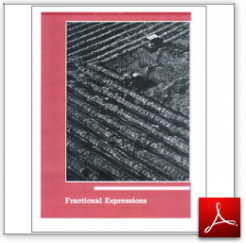Algebra Math Book - Introductory Algebra Thank you for your continual support.

 The power of mathematics rests in the logic of thinking.ID: Chapter06
Price: 9.95

# Algebra Math book - Introductory Algebra - Chapter 6 - Fractional Expressions

## Chapter 6 of this algebra math book has 9 sections.

To get started, you might like to take a free test just to see what areas you might need to study.  Take the test.

 Sec6.1 Section6.1-Algebraic Fractions Sec6.2 Section6.2-Multiplication Sec6.3 Section6.3-Division Sec6.4 Section6.4-Sum and Difference of Like Fractions Sec6.5 Section6.5-Greatest Common Factors and Least Common Multiples Sec6.6 Section6.6-Sum and Difference of Unlink Fractions Sec6.7 Section6.7-Complex Fractions Sec6.8 Section6.8-Fractional Equations Sec6.9 Section6.9-Literal Equations

For a complete listing of the objectives in this chapter visit the List of Objectives.

This section of my algebra math book, Introductory Algebra, also includes in the download:

• Cover Sheet